# US8319855B2 - Method, apparatus and system for image acquisition and conversion - Google Patents

## Info

Publication number
US8319855B2
US8319855B2 US12/831,712 US83171210A US8319855B2 US 8319855 B2 US8319855 B2 US 8319855B2 US 83171210 A US83171210 A US 83171210A US 8319855 B2 US8319855 B2 US 8319855B2
Authority
US
United States
Prior art keywords
image
acquisition
light intensity
samples
spatially
Prior art date
Legal status (The legal status is an assumption and is not a legal conclusion. Google has not performed a legal analysis and makes no representation as to the accuracy of the status listed.)
Active, expires
Application number
US12/831,712
Other versions
US20110176019A1 (en
Inventor
Feng Yang
Yue Lu
Martin Vetterli
Current Assignee (The listed assignees may be inaccurate. Google has not performed a legal analysis and makes no representation or warranty as to the accuracy of the list.)
Rambus Inc
Original Assignee
Rambus Inc
Priority date (The priority date is an assumption and is not a legal conclusion. Google has not performed a legal analysis and makes no representation as to the accuracy of the date listed.)
Filing date
Publication date
Priority to US29621310P priority Critical
Priority to US30263810P priority
Application filed by Rambus Inc filed Critical Rambus Inc
Priority to US12/831,712 priority patent/US8319855B2/en
Assigned to ECOLE POLYTECHNIQUE FEDERALE DE LAUSANNE reassignment ECOLE POLYTECHNIQUE FEDERALE DE LAUSANNE ASSIGNMENT OF ASSIGNORS INTEREST (SEE DOCUMENT FOR DETAILS). Assignors: LI, YUE, VETTERLI, MARTIN, YANG, FENG
Publication of US20110176019A1 publication Critical patent/US20110176019A1/en
Assigned to RAMBUS INC. reassignment RAMBUS INC. ASSIGNMENT OF ASSIGNORS INTEREST (SEE DOCUMENT FOR DETAILS). Assignors: ECOLE POLYTECHNIQUE FEDERALE DE LAUSANNE (EPFL)
Publication of US8319855B2 publication Critical patent/US8319855B2/en
Application granted granted Critical
Active legal-status Critical Current

• 238000006243 chemical reactions Methods 0.000 title claims abstract description 12
• 238000001914 filtration Methods 0.000 claims abstract description 27
• 238000005070 sampling Methods 0.000 claims abstract description 21
• 239000011159 matrix materials Substances 0.000 claims description 25
• 238000007476 Maximum Likelihood Methods 0.000 claims description 14
• 238000005457 optimization Methods 0.000 claims description 7
• 230000002123 temporal effects Effects 0.000 claims description 7
• 238000005259 measurements Methods 0.000 claims description 6
• 206010034960 Photophobia Diseases 0.000 claims 2
• 239000000562 conjugates Substances 0.000 claims 1
• 238000000034 method Methods 0.000 description 13
• 238000004590 computer program Methods 0.000 description 4
• 230000000875 corresponding Effects 0.000 description 4
• 238000010586 diagram Methods 0.000 description 3
• 230000000694 effects Effects 0.000 description 3
• 230000004044 response Effects 0.000 description 3
• 238000003384 imaging method Methods 0.000 description 2
• 230000003287 optical Effects 0.000 description 2
• 230000001131 transforming Effects 0.000 description 2
• 230000004075 alteration Effects 0.000 description 1
• 238000004458 analytical method Methods 0.000 description 1
• 238000004587 chromatography analysis Methods 0.000 description 1
• 229920003013 deoxyribonucleic acid Polymers 0.000 description 1
• 238000001514 detection method Methods 0.000 description 1
• 238000010191 image analysis Methods 0.000 description 1

## Images

•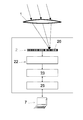•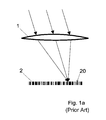•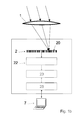•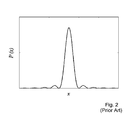•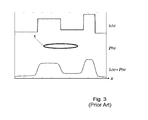•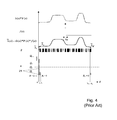•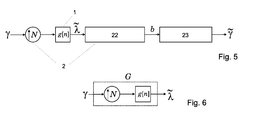•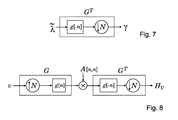•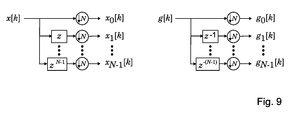•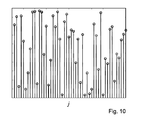•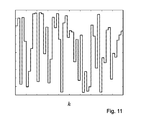•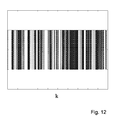•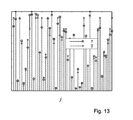•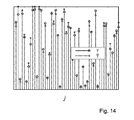## Classifications

• HELECTRICITY
• H04ELECTRIC COMMUNICATION TECHNIQUE
• H04NPICTORIAL COMMUNICATION, e.g. TELEVISION
• H04N5/00Details of television systems
• H04N5/222Studio circuitry; Studio devices; Studio equipment ; Cameras comprising an electronic image sensor, e.g. digital cameras, video cameras, TV cameras, video cameras, camcorders, webcams, camera modules for embedding in other devices, e.g. mobile phones, computers or vehicles
• H04N5/225Television cameras ; Cameras comprising an electronic image sensor, e.g. digital cameras, video cameras, camcorders, webcams, camera modules specially adapted for being embedded in other devices, e.g. mobile phones, computers or vehicles
• H04N5/232Devices for controlling television cameras, e.g. remote control ; Control of cameras comprising an electronic image sensor
• H04N5/23229Devices for controlling television cameras, e.g. remote control ; Control of cameras comprising an electronic image sensor comprising further processing of the captured image without influencing the image pickup process
• HELECTRICITY
• H04ELECTRIC COMMUNICATION TECHNIQUE
• H04NPICTORIAL COMMUNICATION, e.g. TELEVISION
• H04N5/00Details of television systems
• H04N5/30Transforming light or analogous information into electric information
• H04N5/335Transforming light or analogous information into electric information using solid-state image sensors [SSIS]
• H04N5/341Extracting pixel data from an image sensor by controlling scanning circuits, e.g. by modifying the number of pixels having been sampled or to be sampled
• H04N5/347Extracting pixel data from an image sensor by controlling scanning circuits, e.g. by modifying the number of pixels having been sampled or to be sampled by combining or binning pixels in SSIS

## Abstract

A method for image acquisition and conversion includes low-pass filtering an image by an acquisition lens, producing from the low-pass filtered image, an up-sampled image with a first resolution with an up-sampling factor using a image sensor. The up-sampled image is converted into a multi-level image with a second resolution lower than the first resolution with an image processing circuit. The converting depends on the low-pass filtering of the lens and on the up-sampling factor. The method is adapted to gigapixel sensors and convention image sensors.

## Description

REFERENCE DATA
This application claims priority of US2010/61296213, filed on Jan. 19, 2010 and of US2010/61302638, filed on Feb. 9, 2010, the contents whereof are hereby incorporated.
FIELD OF THE INVENTION
The present invention concerns a method for image acquisition and conversion. The present invention concerns also an image acquisition apparatus and a computer-program product.
DESCRIPTION OF RELATED ART
A simplified architecture of a camera or other imaging system is shown on FIG. 1 a. The camera comprises a lens 1 that focuses the incident light onto the plane of an image sensor 2 comprising a plurality of pixels 20 in a row or in an array. Each pixel 20 in the image sensor collects photons, and converts them to analog electrical signals, which are then quantized by a quantizer, such as an A/D converter, into 1, 8 or 12 bits for example. The number of photons hitting the pixel can be modeled by a Poisson process. The quantizers can be provided in each pixel or in a read-out circuit shared by a plurality of pixels.
The image sensor can be considered as a sampling device, which samples the integration function of the light intensity field during a given exposure time and over a space region. One of the most important parameter in a camera is the size of the pixels, which determines the spatial sampling interval.
Due to the diffraction limit, Rayleigh criterion gives the minimum spatial resolution of an ideal lens. The impulse response of the lens 1 is a point spread function (PSF), P(x) shown in FIG. 2. Because the lens has aberrations, and because there is diffraction even with an ideal lens, the point spread function is not a Dirac delta function; instead, the lens acts as a low-pass filter with a minimum spatial resolution larger than zero.
A model of the lens is shown as example in FIG. 3. The light intensity field before the lens is λ(x), where x denotes space index. Due to the low-pass effect of the lens, the light intensity field after the lens is bandlimited and equal to λ(x)*P(x), (* being the convolution operator).
Thanks to the scaling effects in CMOS technology, the state of the art pixel size used in consumer camera and other imaging equipments is often smaller than the minimum spatial resolution of the lens. In this case, the image sensor acts as an oversampling device and produces more samples than what would be required by the bandwidth of the image signal after bandlimitation by the lens.
Moreover, so-called gigapixel camera (or gigapixel digital film) are also known in which a massive oversampling factor is used. The image sensor of gigapixel camera have a non-linear response, similar to a logarithmic function, which make them very suitable for acquiring high dynamic range scenes. Moreover, gigapixel cameras usually have a single photon detector at each pixel, which reduce exposure time in low light conditions, due to the highly sensitive photon detection mechanism. Gigavision cameras can be used for taking videos or photos or, in general, any kind of image, including for example medical images using X-rays or other wavelengths instead of light.
Usually, the pixels in a gigapixel camera have a I-bit quantizer for converting the output of each pixel into binary values (black or white). The image output by the gigapixel sensor thus has a very high spatial resolution but only 2 levels at each pixels, for example only two grey levels. Therefore, a processing circuit is required in order to convert the high resolution, binary output of the gigapixel sensor into an image signal with a lower resolution but with more grey levels.
According to one aspect, the present invention thus relates to image conversion, in particular to methods for the reconstruction of an estimate of a multilevel image based on an oversampled digital signal. In some embodiments, the present invention relates to the conversion of a high resolution binary image signal into a lower resolution multilevel signals. In another embodiments, the present invention also relates to the conversion of a multilevel image signal into another signal with a different number of levels at each or some pixels, and/or with a different resolution. All embodiments can be used for the conversion of 1D, 2D or N-D image signals output by any image sensor.
FIG. 4 illustrates schematically an image sensor 2. The effect of this sensor is to sample the incoming light that passes through the lens 1. The number of electrons Sk generated by the kth pixel depends on the number of photons impinging on this pixel. For example, if the quantum efficiency of the pixel is ‘1’, the number of electrons generated by a pixel 20 is equal to the number of photons received by this pixel.
During an exposure time τ, and pixel width Δx, the number of electrons Sk generated by the kth pixel obeys a Poisson distribution with parameters {tilde over (λ)}k which is the average number of electrons generated by the pixel during the exposure time. This distribution can be written as:
$P ⁡ [ S k = i ] = ⅇ - λ ~ k ⁢ λ ~ k i i !$
As illustrated on FIG. 4, the value delivered by the image sensor 2 is a convolution of the signal after the lens λ(x)*P(x) with a kernel function ƒ(x), followed by a sampling of the resulting function {tilde over (λ)}(x) at
$x = x k - 1 + x k 2 .$
A quantizer is then used to quantize the number of electrons and produces a pixel value Bk which is also a random variable:
${ ℙ ⁡ [ B k = 0 ] = ∑ i = 0 Q 1 - 1 ⁢ ⅇ - λ ~ k ⁢ λ ~ k i i ! ℙ ⁡ [ B k = 1 ] = ∑ i = Q 1 Q 2 - 1 ⁢ ⅇ - λ ~ k ⁢ λ ~ k i i ! … ℙ ⁡ [ B k = L - 1 ] = ∑ i = Q L - 1 + ∞ ⁢ ⅇ - λ ~ k ⁢ λ ~ k i i ! , k = 1 , 2 , … ⁢ , K .$
The starting position of the kth pixel is xk-1, and the ending position is xk. Therefore, the estimation {tilde over (λ)}k of the light received by the pixel is:
$λ ~ k = ∫ x k - 1 x k ⁢ τ ⁢ ⁢ λ ⁡ ( x ) * P ⁡ ( x ) ⁢ ⅆ x = τ ⁢ ⁢ λ ⁡ ( x ) * P ⁡ ( x ) * f ⁡ ( x ) * δ ⁡ ( x - x k - 1 + x k 2 ) ,$
After quantization, the image sensor 2 produces a set of numerical values B=[B1, B2, . . . , BK]T, b=[b1, b2, . . . , bK]T is a realization of the random variable B.
One aim of the present invention is thus to produce an estimate of the light intensity field {tilde over (λ)}(x), given those quantized pixel value.
In particular, it is an aim of the present invention to retrieve the light intensity field {tilde over (λ)}(x) based on the output of an oversampling image sensor, i.e., an image sensor with a spatial and/or temporal frequency larger than the Nyquist rate of {tilde over (λ)}(x)
In the special case of a gigapixel sensor, an aim of the invention is to reconstruct a conventional image (with grey levels) using the binary, higher resolution image output by the sensor.
Reconstruction methods for reconstructing an image from quantized measurements taken by an oversampling camera, such as a gigapixel camera, are known in the prior art. For example, it is known to add or average signals delivered by adjacent pixels in blocks. In the case of a gigapixel camera, it has been suggested for example to produce a multivalued signal that indicates grey levels by summing the binary values B within blocks of adjacent pixels. Other methods based on a low-pass filtering and downsampling the output of the image sensor are also known. This processing is usually done in the readout circuit of the image sensor, or could be done by any digital image processing system in the camera or in a computer or other processing system that receives and process the captured images.
A problem of this prior art approach is that the performance of reconstructing images by low-pass filtering and down-sampling is not good, or at least not optimal.
One difficulty in prior art image sensor is to determine the thresholds Q1 used by the quantizer to distinguish between levels. For example, when the light intensity is high, a gigapixel camera with a large threshold Q1 works better than one with a small threshold. When the light intensity is small, a gigavision camera with a small threshold Q1 works better than one with a large threshold. The same difficulties apply also with image sensors producing multilevel images.
Therefore, there is a need for an improved image acquisition and reconstruction method and apparatus that deliver a better quality of image and that produce a better estimate of the incoming light field based on quantized values generated by the image sensor.
There is also a need to improve the speed of the reconstruction algorithm for reconstructing an image from quantized measurements taken by the camera.
There is also a need for a reconstruction method where the computing complexity does not significantly increase when an image is captured under multiple exposures or with massive spatial oversampling.
BRIEF SUMMARY OF THE INVENTION
The reconstruction method and apparatus of the invention is based in part on novel uses of the sampling theory. This theory tells us that we can perfectly reconstruct an estimated light intensity field {tilde over (λ)}(x) from samples γj, j=1, 2, . . . , J at Nyquist rate:
$λ ~ ⁡ ( x ) = ∑ j = 1 J ⁢ γ j ⁢ ϕ ( x - x j ⁢ ) ,$
where γj, j=1, 2, . . . , J are samples of the estimated light intensity field {tilde over (λ)}(x) at Nyquist rate, φ(x) is a kernel function, and xj is the sampling position for the jth pixel. The oversampling rate is
$N = K J .$
Taking into account the sampling position xk, k=1, 2, . . . , K, the previous expression can be written in digital form as:
$λ ~ k ≈ ∑ j = 1 J ⁢ γ j ⁢ ϕ N ⁡ ( x k - x j ) .$
We use the symbol “≈” in the above equation since the kernel function is often not the ideal one, but only an approximation, based on the assumption that when the pixel size Δx is changed by a factor N, the kernel function needs only to be changed by this scaling factor.
Therefore, according to an aspect of the invention, the above mentioned aims are achieved by means of a method where the conversion of the image signal is not just a series of low-pass filtering and downsampling operations as in the prior art; instead, this conversion is considered as a reconstruction of a signal from digital samples values, for example reconstruction of a signal from digital values taken at a sampling rate above the Nyquist rate, and taking into account properties of the whole optical and electronic system, in particular properties of the lens.
The above mentioned aims are also achieved by means of a method for image acquisition and conversion comprising
• low-pass filtering (or more generally transforming with a transfer function) an image by an acquisition lens,
• producing from said low-pass filtered image an up-sampled image with a first resolution with an up-sampling factor using a image sensor,
• converting said up-sampled image into a multi-level image with a second resolution lower than said first resolution with an image processing circuit
wherein said converting step depends on said low-pass filtering of said lens and on said up-sampling factor.
The reconstruction depends on the transfer function of the lens and on the oversampling factor, thus resulting in an optimization of the whole system and in an improved image quality.
The output delivered by the reconstruction process is typically a multi-level image, i.e., an image with more than two different possible values at each pixel, such as a grayscale or color image.
In one embodiment, the up-sampled image is produced with a gigapixel image sensor that outputs a binary up-sampled image.
In one embodiment, the sensor quantizer comprises a spatially-varying arrangement. For example, in the 1-bit quantizer case, one group of pixels on the sensor could have a threshold Q1=1, and the rest of the pixels a higher threshold, or various different thresholds.
Advantageously, the conversion into a multi-level image is done with a method and circuit that implements a maximum likelihood estimation method.
According to one aspect, the invention is based on the finding that the negative log-likelihood function is a convex function; this is true in particular when the threshold Q of the quantizer is “1”, but also when the threshold is different from “1”, and with quantizers having a plurality of thresholds for producing multilevel measurement values B. Therefore, optimal solution can be achieved using convex optimization.
In one embodiment, at least two exposures are provided for each image acquired, thus resulting in temporal oversampling and in even more samples used for each image. Methods are described that allow using multiple exposure without substantial increase of the computational complexity.
According to an aspect, the reconstruction method uses a maximum likelihood method based on filter bank techniques for computing the gradient and the multiplication of a vector and Hessian matrix of the negative log-likelihood function. The use of filter bank techniques results in fast processing.
Advantageously, a polyphase representation or another improved representation of the signals and operators is used in order to increase the computing speed.
An important advantage of the method according to the invention is to allow a reconstruction of an image exploiting and depending on the low-pass filtering function of the lens and the up-sampling function of the gigavision sensor.
Advantageously, the reconstruction of the image from the measurements output by the sensor can be done by DSP elements, by FPGA components, by a microprocessor or microcontroller inside a camera or in a computer. For example, the maximum likelihood estimation used within the method can be carried out by means among this list, or by any other suitable hardware or software means.
For this purpose, a program of image elaboration during the treatment of image takes into account the sensor and the lens of the camera. In another embodiment, the reconstruction is done by an image processing apparatus outside of the camera, for example by an image processing software executed by a computer or IT equipment that receives and processes image files output by the camera. The invention also relates to a computer program product that tangibly and permanently stores a computer program for causing a processing system to perform the method described in this application.
BRIEF DESCRIPTION OF THE DRAWINGS
The invention will be better understood with the aid of the description of an embodiment given by way of example and illustrated by the Figures, in which:
FIG. 1 a shows a simplified architecture of a camera. The incident light is focused by the lens and then impinges on the image sensor. After that, each pixel in the image sensor converts the received photons to electrons.
FIG. 1 b shows a simplified architecture of an image acquisition apparatus comprising a camera and additional image processing means.
FIG. 2 shows an example of point spread function of the lens.
FIG. 3 shows the model of the lens. The lens is modeled as a linear system with impulse response P(x). The result of a light intensity field λ(x) passing through the lens is λ(x)*P(x).
FIG. 4 shows a model of the image sensor using an L-level quantizer with levels, Q1, Q2, . . . , QL−1 to quantize the number of electrons and to produce the pixel values Bk, k=1, 2, . . . , K.
FIG. 5 shows a example of model for the camera of the invention. γ=[γ1, γ2, . . . , γJ]T are the samples of {tilde over (λ)}(x) at Nyquist rate. N is upsampling factor. g[n] is a low-pass filter. {tilde over (λ)}=[{tilde over (λ)}1, {tilde over (λ)}2, . . . , {tilde over (λ)}K]T are oversampled samples of {tilde over (λ)}(x). B=[B1, B2, . . . , BK]T are the quantized pixel values, b=[b1, b2, . . . , bK]T is a realization of B. {circumflex over (γ)}=[{circumflex over (γ)}1, {circumflex over (γ)}2, . . . , {circumflex over (γ)}J]T are the reconstructed values of {tilde over (λ)}(x).
FIG. 6 shows the upsampling and low-pass filtering operator G.
FIG. 7 shows the operator GT for low-pass filtering and downsampling.
FIG. 8 shows a diagram from computing L(γ)'s Hessian matrix Hv times a vector v. g[n] and g[−n] are low-pass filters.
FIG. 9 shows the polyphase representation of a sequence x[k] (left) and a filter g[k] (right).
FIG. 10 shows an example of a 1-D synthesized signal γ.
FIG. 11 shows an example of an upsampled and low-pass filtered signal {tilde over (λ)} using an oversampling factor N=100, and an exposure time τ=1.
FIG. 12 shows an example of a binary sequence generated by the camera with threshold Q1=1, an oversampling factor N=100, and totally exposure time τ=1.
FIG. 13 shows an example of a 1-D synthesized signal γ, as well as the estimated signal {circumflex over (γ)}, using a threshold a Q1=1, an oversampling factor N=100, a number of exposures M=1, and an exposure time τ=1.
FIG. 14 shows an example of a 1-D synthesized signal γ, as well as the estimated signal {circumflex over (γ)}, using a threshold Q1=1, an oversampling factor N=20000, a number of exposures M=1 and an exposure time τ=1.
In another embodiment, the quantizer is an L-level quantizer 4 with a plurality of levels Q1, Q2, . . . , QL−1; the thresholds between the levels can be equidistant or not, and the quantized produces multilevel pixel values Bk, k=1, 2, . . . , K indicating level of grays for each of the K pixels. As will be described later, different quantizers with different number of thresholds, and/or with different distances between the thresholds, can be associated with one single image sensor.
Reference 23 on FIG. 1 b shows a processing circuit for processing the quantized pixel value B delivered by the quantizer(s) 22. The processing circuit may be built around a microprocessor, a microcontroller, a digital processing circuit, a FPGA, an asic, etc. The processing circuit is connected to a memory, for example a semi permanent memory 25 such as a Flash memory for example, for storing software executed by the processing circuit (bios) and processed images delivered by the processing circuit 23. The processing circuit executes program or functions for converting the image signals delivered by the image sensor 22 into lower-resolution image files, using reconstruction methods described below. Those reconstruction methods can also be executed, entirely or in part, by programs executed outside of the camera, for example in a personal computer 7, workstation, server or any other IT system able to receive and process oversample samples produced by the image sensor.
Since the reconstruction depends on the transfer function of the lens and on the oversampling factor used by the image sensor, those parameter need to be known by the processing circuit 23 that perform the reconstruction. If the lens is not integral with this processing circuit, the type of lens which is used, or its transfer function, can be indicated as metadata in the image file, or input by the user, or retrieved from a knowledge database for instance or a priori known. The oversampling factor can usually be retrieved from the image files, for example by determining the number of samples b.
FIG. 5 is another block diagram of the apparatus of the invention. The light intensity field γ=[γ1, γ2, . . . , γJ]T is spatially (and/or temporally) upsampled by a factor N by the camera sensor 2 and filtered by a low-pass filter g[n] corresponding to the lens 1, thus producing oversampled samples of {tilde over (λ)}(x), {tilde over (λ)}=[{tilde over (λ)}1, {tilde over (λ)}2, . . . , {tilde over (λ)}K]T. The relation between the light intensity field {tilde over (λ)} at the output of the image sensor and the light intensity field γ before the lens can be written as {tilde over (λ)}=Gγ, where G=[g1, g2, . . . , gK]T is a K×J matrix representing the upsampling and low-pass filtering operator, as shown in FIG. 6.
A quantized pixel value B is then generated by the quantizers 22. Finally a reconstruction algorithm is executed by digital processing means 23 in the camera or in a digital processing system to obtain an estimate of the light intensity field {circumflex over (γ)}=[{circumflex over (γ)}1, {circumflex over (γ)}2, . . . , {circumflex over (γ)}J]T.
The aim of the reconstruction is to compute the estimate {circumflex over (γ)} of the light intensity field. According to one aspect of the invention, the reconstruction method carried out by the digital processing means 23 uses a maximum likelihood estimator (MLE), or other maximum likelihood estimating means, for solving the reconstruction problem and for computing {circumflex over (γ)}:
$γ ^ = ⁢ arg ⁢ ⁢ max γ ⁢ ⁢ ℙ ⁡ ( B ; γ ) = ( 1 ) ⁢ ⁢ arg ⁢ ⁢ max γ ⁢ ∏ k = 1 K ⁢ ∑ l = 0 L - 1 ⁢ δ ⁡ ( B k - l ) ⁢ ℙ ⁡ ( B k = l ; λ ~ k ) = ( 2 ) ⁢ ⁢ arg ⁢ ⁢ max γ ⁢ ⁢ ln ⁡ ( ∏ k = 1 K ⁢ ∑ l = 0 L - 1 ⁢ δ ⁡ ( B k - l ) ⁢ ℙ ⁡ ( B k = l ; λ ~ k ) ) = ( 3 ) ⁢ ⁢ arg ⁢ ⁢ min γ - ∑ k = 1 K ⁢ ln ⁡ ( ∑ l = 0 L - 1 ⁢ δ ⁡ ( B k - l ) ⁢ ℙ ⁡ ( B k = l ; λ ~ k ) ) = ⁢ arg ⁢ ⁢ min γ ⁢ ℒ ⁡ ( γ )$
Those relations are based on the following findings: in (1), we use the independence of each pixel when given γk, in (2) we use the fact that the logarithm function ln does not change the solution of a maximization problem, and in (3) we use the property that the solution of maximizing a function is equal to minimize the corresponding negative function.
For each pixel k, the negative log-likelihood function L(γ) can be written as
$L k ⁢ ⁢ ( γ ) = - ln ⁡ ( ∑ l = 0 L - 1 ⁢ δ ⁡ ( B k - l ) ⁢ P ⁡ ( B k = l ; λ ~ k ) ) , then ⁢ ⁢ L ⁡ ( γ ) = ∑ k = 1 K ⁢ L k ⁡ ( γ ) .$
It can be demonstrated that L(γ) is a convex function, even if the quantizer is a multi-level quantizer. A demonstration can be found in the Appendix.
In addition to or instead of the spatial oversampling, it is also possible to perform a temporal oversampling by taking M pictures during each time period [0,τ]. In this example, all pictures are taken with the same exposure time
$τ M ;$
different exposure times can also be considered.
$λ ~ ~ ⁡ ( x ) = λ ~ ⁡ ( x ) M = τ M ⁢ λ ⁡ ( x ) * P ⁡ ( x ) * f ⁡ ( x ) ,$
which is the same for each exposure. So we scale down the original parameter function by a factor of M. Then
$γ ~ = γ M , and ⁢ ⁢ λ ~ ~ = [ λ ~ ~ 1 , λ ~ ~ 2 , … ⁢ , λ ~ ~ k ] T = λ ~ M .$
Let {tilde over (B)}[B1, B2, . . . , BM], where Bm=[Bm1, Bm2, . . . , BmK]T, m=1, 2, . . . , M is the output pixel values during mth exposure, and Bmk, k=1, 2, . . . , K is the pixel value of kth pixel, during the mth exposure. The maximum likelihood estimator (MLE) to estimate γ is
$γ ^ = ⁢ arg ⁢ ⁢ max γ ⁢ ℙ ⁡ ( B ~ ; γ ) = ( 1 ) ⁢ ⁢ arg ⁢ ⁢ max γ ⁢ ∑ m = 1 M ⁢ ∑ k = 1 K ⁢ ∑ l = 0 L - 1 ⁢ δ ⁡ ( B mk - l ) ⁢ ℙ ⁡ ( B mk = l ; λ ~ ~ k ) = ⁢ arg ⁢ ⁢ max γ ⁢ ∑ m = 1 M ⁢ ∑ k = 1 K ⁢ ∑ l = 0 L - 1 ⁢ δ ⁡ ( B mk - l ) ⁢ ℙ ⁡ ( B mk = l ; λ ~ k M ) = ( 2 ) ⁢ ⁢ arg ⁢ ⁢ max γ ⁢ ln ⁡ ( ∑ m = 1 M ⁢ ∑ k = 1 K ⁢ ∑ l = 0 L - 1 ⁢ δ ⁡ ( B mk - l ) ⁢ ℙ ⁡ ( B mk = l ; λ ~ k M ) ) = ( 3 ) ⁢ ⁢ arg ⁢ ⁢ min γ - ∑ m = 1 M ⁢ ∑ k = 1 K ⁢ ln ⁡ ( ∑ l = 0 L - 1 ⁢ δ ⁡ ( B mk - l ) ⁢ ℙ ⁡ ( B mk = l ; λ ~ k M ) ) ,$
The estimator thus uses the following findings: in (1), the relation is based on the independence of each pixel when given λk, in (2) the estimator uses the fact that ln does not change the solution of a maximization problem, and in (3) the estimator uses use the property that the solution of maximizing a function is equal to minimize the corresponding negative function.
Thus, according to previous equation,
$γ ^ = arg ⁢ ⁢ min γ ⁢ L ~ ⁡ ( γ ) ,$
where {tilde over (L)}(γ) is the negative log-likelihood function,
$L ~ ⁡ ( γ ) = - ∑ m = 1 M ⁢ ∑ k = 1 K ⁢ ln ⁡ ( ∑ l = 0 L - 1 ⁢ δ ⁡ ( B mk - l ) ⁢ P ⁡ ( B mk = l : λ ~ k M ) ) , γ ∈ R + J .$
$Let ⁢ ⁢ L ~ mk ⁡ ( γ ) = - ln ⁡ ( ∑ l = 0 L - 1 ⁢ δ ⁡ ( B mk - l ) ⁢ P ⁡ ( B mk = l ; λ ~ k M ) ) , then$ $L ~ ⁡ ( γ ) = ∑ m = 1 M ⁢ ∑ k = 1 K ⁢ L ~ mk ⁡ ( γ ) .$
In multiple successive exposures, as in the case of single exposure, it can be demonstrated that {tilde over (L)}(γ) is a convex function. (see Appendix). Therefore, since the negative log-likelihood function is a convex function, the estimator can use any method for solving a convex optimization problem, like interior-point method, trust-region method, or Newton's method for example. Advantageously, the method is selected so as to reduce the computing time and power required to find the solution.
In one embodiment, the estimator uses the following modified Newton's method for estimating the incoming light in the one exposure case. The one skilled in the art can adapt this method to the multiple exposures case. A possible pseudo codes is as follows:
 Pseudocode for Modified Newton's method given a starting point γ0 ε dom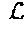(γ), dom(γ) is the domain of function(γ), tolerance ε = 10−5. repeat 1. Compute the Newton step and decrement. Calculate ∇(γ), quit if ||∇(γ)|| · ε Δγ := −∇2(γ)−1∇(γ); θ2 := −∇L (γ)γ Δγ, quit if θ2/2 · ε. 2. Line search. Choose step size φ by backtracking line search. given a descent direction Δγ for(γ) α = 0.25, β = 0.5, φ := 1, while γ + φΔγ ∉ domL(γ), if φ < 10−5, find position set I, that γi ≧ γmin or γi · γmax, i ε I if I is not empty, Δγj = 0, j ε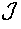− I where= {1,2, . . . ,}. else quit. else φ := βφ while(γ + φΔγ) >(γ) + αφ∇Δγ, φ := βφ 3. Update. γ := γ + φΔγ.
A problem is that the size of the Hessian matrix ∇2L(γ) is very large, so that computing the inverse of this matrix requires a lot of processing power and processing time. According to one aspect, in order to reduce this computing time, the system is programmed so as to perform a conjugate gradients method and directly compute ∇2L(γ)−1∇L(γ).
In most known methods for solving convex optimization problem, like the Newton's method, the interior-point method or the trust-region method, it is needed to provide the negative log-likelihood function's gradient, and the Hessian matrix multiplication with a vector.
The method used in the method of the invention is also based on the finding that the gradient of the negative log-likelihood function
$L ⁡ ( γ ) ⁢ ⁢ is ⁢ ⁢ ∇ L ⁡ ( γ ) = G T ⁡ [ ∂ L 1 ⁢ ⁢ ( γ ) ∂ λ ~ 1 , ∂ L 2 ⁡ ( γ ) ∂ λ ~ 2 , … ⁢ , ∂ L K ⁡ ( γ ) ∂ λ ~ K ] T ,$
and the Hessian matrix of L(γ) is H=GT AG, where,
$A = ( ∂ 2 ⁢ ℒ 1 ⁡ ( γ ) ∂ 2 ⁢ λ ~ 1 0 … 0 0 ∂ 2 ⁢ ℒ 2 ⁡ ( γ ) ∂ 2 ⁢ λ ~ 2 … 0 ⋮ ⋮ ⋱ ⋮ 0 0 … ∂ 2 ⁢ ℒ κ ⁡ ( γ ) ∂ 2 ⁢ λ ~ κ ) .$
A problem with the above two equations is that if the upsampling factor is large, the matrix G is big and huge storage space would be required to store it.
However, the operator G corresponds to the lens and to the upsampling part of the image sensor, and there is no requirement for storing the whole matrix. We only need to know the parameters of the upsampling and low-pass filtering operator, i.e., the upsampling factor N and the coefficients of the low-pass filter g[n]. The coefficients of the low-pass filter of the lens can be stored in a storage of the lens, and transmitted to the processing means that make the transformation. In another embodiment, those coefficients are a priori known by the processing means, for example if the processing means know in advance the properties of the lens that is used. In yet another embodiment, those properties are retrieved by the processing means based on an identification of the type of lens received by the processing means.
We can write G=LU, where L indicates low-pass filtering operator and U denotes upsampling operator. Then GT=(LU)T=UT LT=DR, where D is the matrix notation for downsampling operator and R is the matrix notation for low-pass filtering operator. The downsampling factor of D is equal to the upsampling factor of U. If the filter coefficients of L is g[n], then the filter coefficient of R is g[−n]. In the case of symmetric low-pass filter, the filter coefficients of L and R are the same. FIG. 7 illustrates the matrix for low-pass filtering and downsampling operator GT
The gradient of L(γ) can be computed by first low-pass filtering the vector
$[ ∂ L 1 ⁡ ( γ ) ∂ λ ~ 1 , ∂ L 2 ⁡ ( γ ) ∂ λ ~ 2 , … ⁢ , ∂ L K ⁡ ( γ ) ∂ λ ~ K ] T ,$
then downsampling it by the factor N.
The negative log-likelihood function's Hessian matrix H times a vector v is Hv=GT AGv. FIG. 8 shows the diagram for computing the above equation. The vector v is upsampled with upsampling factor N, then low-pass filter it using a filter g[n].
Since the matrix A is a diagonal matrix, the multiplication of A and vector Gv is equal to the elementwise multiplication of the diagonal of A and Gv. After that, the processing means low-pass filters the obtained vector with a filter of g[−n] and downsamples by a factor of N to get Hv.
Therefore, the processing means can reconstruct the image signal from the measured samples.
According to one aspect of the invention, in order to further increase the speed of the optimization process, a polyphase representation can be used to reduce the computing time of the upsampling and low-pass filtering operator. Different polyphase representations can be defined for a sequence and for a filter.
A 1-D sequence x[k] or filter g[k] can be decomposed into N polyphase components, defined as,
$x i ⁡ [ k ] ⁢ = Δ ⁢ x ⁡ [ Nk + i ] , g i ⁡ [ k ] ⁢ = Δ ⁢ g ⁡ [ Nk - i ] , i = 0 , 1 , … ⁢ , N ⁢ ⁢ ¡1 .$
FIG. 9 schematically illustrates a polyphase representation of a sequence x[k] (left part) and of a filter g[k] (right part). We can also compute this polyphase representation in the z-domain:
$X ⁡ ( z ) = ∑ n = 0 N - 1 ⁢ z - n ⁢ X n ⁡ ( z N ) , and ⁢ ⁢ G ⁡ ( z ) = ∑ n = 0 N - 1 ⁢ z n ⁢ G n ⁡ ( z N ) .$
Let Y(z) be the z transform of the sequence y[k], which is the output when we implement the low-pass filtering and downsampling operator on a signal x[k], then we have
$Y ⁡ ( z ) = ∑ n = 0 N - 1 ⁢ H n ⁡ ( z ) ⁢ X n ⁡ ( z ) .$
This means that the process for the implementation of the operator on a sequence x[k] is decomposing the filter and sequence into N polyphase components, filtering the nth polyphase component of the sequence separately with the corresponding nth polyphase component of the filter, and summing all the filtered results to generate y[k].
During this process, the processing means avoid to compute the sequence value which will be discarded during the downsampling process, therefore the computing time can be saved.
If g[k] is decomposed using the definition of polyphase representation for a sequence, then
$G ⁡ ( z ) = ∑ n = 0 N - 1 ⁢ z - n ⁢ H n ⁡ ( z N )$
and the output of the implementing the upsampling and low-pass filtering operator on x[k] can be written as
$( Y 0 ⁡ ( z ) Y 1 ⁡ ( z ) ⋮ Y N ⁡ ( z ) ) = ( G 0 ⁡ ( z ) G 1 ⁡ ( z ) ⋮ G N ⁡ ( z ) ) ⁢ X ⁡ ( z ) ,$
where Yn(z) is the z transform of the nth polyphase component of the output sequence y[k].
Using this method, the processing means avoid to compute the multiplication of the filter coefficient and the “0”s generated during the upsampling process, and thus increase the speed by a factor of N.
Analysis have also shown that in the 1-bit quantizer case, a lower threshold Q1 leads to small estimation errors in low light situations, but does not perform well in high light intensity regions, for which a higher threshold is more suitable.
To address the problem of a suitable threshold, in one embodiment the sensor quantizer has a spatially-varying arrangement. For instance, in one embodiment using a 1-bit quantizer, a first group of pixel on the sensor has a threshold Q1=1, while other have a higher threshold. Similarly, in embodiment having n-bits quantizers, a plurality of different thresholds can be used for various pixels of the same chip. It is also possible to vary the threshold in time, or depending on lighting conditions.
The two types of pixels can be spatially-interlaced according to a given pattern. It can be shown that in this case, the negative log-likelihood function is still a convex function. All the previous techniques can thus be used.
The arrangement of different types of pixels (with different thresholds) on a given sensor can be designed to achieve an optimal pattern and arrangements of different threshold values, achieving best signal-to-noise ratio.
In order to design an optimal pattern, the following method can be used. If the total number of pixels is N, the maximum threshold value Qmax, and aε[0,amax]. In an example, the pixels only have two different thresholds Q11, and Q12, N1 be the number of pixels have threshold Q11, and N2=N−N1 be the number of pixels have threshold Q12. Then the problem is what is the optimal Q11, Q12, N1, N2 that can maximize argamin SNRmax (Q11, Q12, N1, N2)? This is equal to solve the problem:
$Q 11 , Q 12 , N 1 , N 2 = arg ⁢ ⁢ max Q 11 , Q 12 , N 1 , N 2 ⁢ arg ⁢ ⁢ min a ⁢ SNR max ⁡ ( Q 11 , Q 12 , N 1 , N 2 )$
s.t. aε[0,a max ],Q 11 ,Q 12ε[1,Q max ],N 1 ,N 2ε[0,N],N 1 +N 2 =N,
where
$SNR max ⁡ ( Q 11 , Q 12 , N 1 , N 2 ) = a ⁢ R Q 1 2 N 1 ⁢ MP Q 1 ⁡ ( ⅇ a N 1 ⁢ M - P Q 1 ) + R Q 2 2 N 2 ⁢ MP Q 2 ⁡ ( ⅇ a N 2 ⁢ M - P Q 2 ) .$
Since this optimal pattern design is only done once, during design of a sensor chip, and the variables range is not large, exhaustive search method can be used to solve this optimization problem.
Here we only consider the case that we have two thresholds, more complex cases that have multiple thresholds, or multi-level quantizer can also be done in the same way.
A simple example is given. When amax=100, Qmax=9, and N=100, M=1. Using the above algorithm, the optimal pattern is that N1=37 pixels have Q11=1, and N2=63 pixels have Q12=9.
Experimental results for 1D and for 2D images have shown that increasing the spatial and/or temporal oversampling factor increase the performance of the apparatus and method when the threshold Q1=1. When the light intensity is large, for small Q1, there is a high probability that the sensor will be saturated, i.e., all the pixel values will be “1”. So when the light intensity is large, we need larger thresholds Q1. When the light intensity is small, for large Q1, there is a high probability that the sensor will have all “0” output, which makes the sensor not sensitive to low light intensity. So when the light intensity is small, we need small Q1. If Q1>2, there exists an optimal (NM)opt for a given γj. The NM larger than (NM)opt will have worse performance.
FIG. 10 shows an example of a 1-D synthesized signal γ. FIG. 11 shows an example of an upsampled and low-pass filtered signal {tilde over (λ)}, using an oversampling factor N=100, and an exposure time τ=1. FIG. 12 shows an example of a binary sequence generated by the camera/image sensor with threshold Q1=1, an oversampling factor N=100, and total exposure time τ=1. FIG. 13 shows an example of a 1-D synthesized signal γ, as well as the estimated signal {circumflex over (γ)}, using a threshold Q1=1, an oversampling factor N=100, a number of exposures M=1, and an exposure time of τ=1. FIG. 14 shows an example of a 1-D synthesized signal γ, as well as the estimated signal {circumflex over (γ)}, using a threshold Q1=1, an oversampling factor N=20000, a number of exposures M=1 and an exposure time τ=1.
The above described methods may be performed by any suitable means capable of performing the operations, such as various hardware and/or software component(s) in a still or video camera, in other image acquisition devices, or in any image processing apparatus, including computer and workstation with suitable image processing applications.
The above described methods and apparatus can be used in consumer image acquisition systems, such as still and video cameras, mobile phone with camera, webcams, etc. Those methods and apparatus are in particular useful for the acquisition of still and video images with a high dynamic range, such as but not limited to high dynamic range photography, low light acquisition (for astronomy or night images), DNA image analysis, chromatography etc.
The various equations and processing steps described in the present application may be performed by a software executed by a general purpose processor or by a digital signal processor (DSP), by an application specific integrated circuit (ASIC), by a field programmable gate array signal (FPGA), by discrete components or any combination thereof. The apparatus may be an image acquisition apparatus, such as a camera comprising a lens, an image processing apparatus in the camera or as a separate equipment (such as a separate computer), or a combination between the two, such as a camera used in combination or sequentially with a computer for acquiring and processing still or video images.
Any steps of a method according to the present application may be embodied in hardware, in a software module executed by a processor, or in a combination of the two. Thus, the invention also relates to a computer program product for performing the operations presented herein. If implemented in software, the functions described may be stored as one or more instructions on a computer-readable medium. Some examples of storage media that may be used include random access memory (RAM), read only memory (ROM), flash memory, EPROM memory, EEPROM memory, registers, a hard disk, a removable disk, other optical disks, or any available media that can be accessed by a computer, a camera or an image acquisition apparatus.
APPENDIX
By applying the chain rule, we can show that:
$∇ ⁢ ℒ ⁡ ( γ ) = ∂ ℒ ⁡ ( γ ) ∂ γ = ∂ λ ~ ∂ γ ⁢ ∂ ℒ ⁡ ( γ ) ∂ λ ~ = G T ⁢ ∂ ∑ k = 1 K ⁢ ⁢ ℒ k ⁡ ( γ ) ∂ λ ~ = G T ⁡ [ ∂ ℒ 1 ⁡ ( γ ) ∂ λ ~ 1 , ∂ ℒ 2 ⁡ ( γ ) ∂ λ ~ 2 , … ⁢ , ∂ ℒ K ⁡ ( γ ) ∂ λ ~ K ] T$
The Hessian Matrix of L(γ)
The Hessian matrix of L(γ) is H=GT AG, where
$A = ( ∂ 2 ⁢ ℒ 1 ⁡ ( γ ) ∂ 2 ⁢ λ ~ 1 0 … 0 0 ∂ 2 ⁢ ℒ 2 ⁡ ( γ ) ∂ 2 ⁢ λ ~ 2 … 0 ⋮ ⋮ ⋱ ⋮ 0 0 … ∂ 2 ⁢ ℒ K ⁡ ( γ ) ∂ 2 ⁢ λ ~ K ) .$
Proof:
According to the chain rule,
$H = ∂ γ ⁢ ( ∂ ℒ ⁡ ( γ ) ∂ γ ) = ∂ λ ~ ∂ γ ⁢ ∂ λ ~ ⁢ ( ∂ ℒ ⁡ ( γ ) ∂ γ ) = G T ⁢ ∂ [ ∂ ℒ 1 ⁡ ( γ ) ∂ λ ~ 1 , ∂ ℒ 2 ⁡ ( γ ) ∂ λ ~ 2 , … ⁢ , ∂ ℒ K ⁡ ( γ ) ∂ λ ~ K ] T ∂ λ ~ ⁢ G = G T ⁡ ( ∂ 2 ⁢ ℒ 1 ⁡ ( γ ) ∂ 2 ⁢ λ ~ 1 0 … 0 0 ∂ 2 ⁢ ℒ 2 ⁡ ( γ ) ∂ 2 ⁢ λ ~ 2 … 0 ⋮ ⋮ ⋱ ⋮ 0 0 … ∂ 2 ⁢ ℒ K ⁡ ( γ ) ∂ 2 ⁢ λ ~ K ) ⁢ G$
Convexity of L(γ)
To prove L(γ) is a convex function, it is sufficient to show that the Hessian matrix of L(γ), H is positive semidefinite. Looking at the Hessian matrix, we need to prove that
$∂ 2 ⁢ L k ⁡ ( y ) ∂ 2 ⁢ λ ~ k ≥ 0 , ⁢ k = 1 , 2 , … ⁢ ⁢ K .$
Let
$P Q ⁡ ( x ) = { ∑ q = 0 Q - 1 ⁢ ⁢ x q q ! , Q ≥ 1 0 , Q ≤ 0 , ⁢ ⁢ R Q ⁡ ( x ) = { x Q - 1 ( Q - 1 ) ! , Q ≥ 1 0 , Q ≤ 0 ,$
To simply the notation, we use PQ to indicate the function PQ(x). The first derivative of PQ is, P′Q=PQ−1. The first derivative of R′Q=RQ−1. We also have the equation that PQ−PQ−1=RQ, and
$P Q ⁡ ( x ) = ∑ q = - ∞ Q ⁢ ⁢ R q ⁡ ( x ) .$
When Bk=0,
$∂ ℒ k ⁡ ( y ) ∂ λ ~ k = - ∂ ln ⁡ ( ℙ ⁡ ( B k = 0 ; λ ~ k ) ) ∂ λ ~ k = - ∂ ln ⁡ ( ⅇ - λ ~ k ⁢ P Q 1 ⁡ ( λ ~ k ) ) ∂ λ ~ k = 1 - P Q 1 ′ P Q 1 = 1 - P Q 1 - 1 P Q 1 = R Q 1 P Q 1 ∂ 2 ⁢ ℒ k ⁡ ( γ ) ∂ 2 ⁢ λ ~ k = ∂ ∂ λ ~ k ⁢ ( R Q 1 P Q 1 ) = R Q 1 ′ ⁢ P Q 1 - R Q 1 ⁢ P Q 1 ′ P Q 1 2 = R Q 1 - 1 ⁢ P Q 1 - R Q 1 ⁢ P Q 1 - 1 P Q 1 2$
If Q1=1, then, RQ 1 −1=0, PQ 1 =1, RQ 1 =1, PQ 1 −1=0, then
$∂ 2 ⁢ L k ⁡ ( γ ) ∂ 2 ⁢ λ ~ k = 0 ≥ 0 ,$
If Q1=2, then, RQ 1 −1=1, PQ 1 =1+{tilde over (λ)}k, RQ 1 ={tilde over (λ)}k, PQ 1 −1=1,
then
$∂ 2 ⁢ L k ⁡ ( γ ) ∂ 2 ⁢ λ ~ k = 1 + λ ~ k - λ ~ k P Q 1 2 = 1 P Q 1 2 ≥ 0.$
If Q1≧3,
$∂ 2 ⁢ ℒ k ⁡ ( γ ) ∂ 2 ⁢ λ ~ k = R Q 1 - 1 ⁢ P Q 1 - R Q 1 ⁢ P Q 1 - 1 P Q 1 2 = R Q 1 - 1 P Q 1 2 ⁢ ( P Q 1 - λ ~ k Q 1 - 1 ⁢ P Q 1 - 1 ) = R Q 1 - 1 P Q 1 2 ⁢ ( ∑ q = 0 Q 1 - 1 ⁢ ⁢ λ ~ k q q ! - λ ~ k Q 1 - 1 ⁢ ∑ q = 0 Q 1 - 2 ⁢ ⁢ λ ~ k q q ! ) = R Q 1 - 1 P Q 1 2 ⁢ ( ∑ q = 0 Q 1 - 1 ⁢ ⁢ λ ~ k q q ! - 1 Q 1 - 1 ⁢ ∑ q = 0 Q 1 - 2 ⁢ ⁢ λ ~ k q + 1 q ! ) = R Q 1 - 1 P Q 1 2 ⁢ ( ∑ q = 0 Q 1 - 1 ⁢ ⁢ λ ~ k q q ! - 1 Q 1 - 1 ⁢ ∑ q = 0 Q 1 - 1 ⁢ ⁢ q ⁢ λ ~ k q q ! ) = R Q 1 - 1 P Q 1 2 ⁢ ∑ q = 0 Q 1 - 1 ⁢ ( 1 - q Q 1 - 1 ) ⁢ λ ~ k q q ! ≥ 0$
and we also have RQ−1PQ−RQPQ−1≧0, when Q≧3 (2).
When 1≦Bk=l≦L−2,
$∂ ℒ k ⁡ ( y ) ∂ λ ~ k = - ∂ ln ⁡ ( ℙ ⁡ ( B k = l ; λ ~ k ) ) ∂ λ ~ k = - ∂ ln ⁡ ( ⅇ - λ ~ k ⁡ ( P Q l + 1 ⁡ ( λ ~ k ) - P Q l ⁡ ( λ ~ k ) ) ) ∂ λ ~ k = 1 - P Q l + 1 ′ - P Q l ′ P Q l + 1 - P Q l = 1 - P Q l + 1 - 1 - P Q l - 1 P Q l + 1 - P Q l = R Q l + 1 - R Q l P Q l + 1 - P Q l ∂ 2 ⁢ ℒ k ⁡ ( γ ) ∂ 2 ⁢ λ ~ k = ∂ ∂ λ ~ k ⁢ ( R Q l + 1 - R Q l P Q l + 1 - P Q l ) = ( R Q l + 1 ′ - R Q l ′ ) ⁢ ( P Q l + 1 - P Q l ) - ( R Q l + 1 - R Q l ) ⁢ ( P Q l + 1 ′ - P Q l ′ ) ( P Q l + 1 - P Q l ) 2 = ( R Q l + 1 - 1 - R Q l - 1 ) ⁢ ( P Q l + 1 - P Q l ) - ( R Q l + 1 - R Q l ) ⁢ ( P Q l + 1 - 1 - P Q l - 1 ) ( P Q l + 1 - P Q l ) 2 .$
Here Ql+1 should be greater than Ql.
If Ql=1, Ql+1=2, then, RQ l+1 −1=R1=1, RQ l −1=R0=0, PQ l+1 =P2=1+{tilde over (λ)}k, PQ l =P1=1,
$R Q l + 1 = λ ~ k , R Q l = R 1 = 1 , P Q l + 1 - 1 = P 1 = 1 , P Q l - 1 = 0 , ⁢ ∂ 2 ⁢ ℒ k ⁡ ( γ ) ∂ 2 ⁢ λ ~ k = ( R Q l + 1 - 1 - R Q l - 1 ) ⁢ ( P Q l + 1 - P Q l ) - ( R Q l + 1 - R Q l ) ⁢ ( P Q l + 1 - 1 - P Q l - 1 ) ( P Q l + 1 - P Q l ) 2 = 1 ( P Q l + 1 - P Q l ) 2 ≥ 0$
If Ql=1, Ql+1≧3, then, RQ l −1=R0=0, PQ l =P1=1, RQ l =R1=1, PQ l −1=0,
$∂ 2 ⁢ ℒ k ⁡ ( γ ) ∂ 2 ⁢ λ ~ k = R Q l + 1 - 1 ⁡ ( P Q l + 1 - 1 ) - ( R Q l + 1 - 1 ) ⁢ P Q l + 1 - 1 ( P Q l + 1 - 1 ) 2 = R Q l + 1 - 1 ⁢ P Q l + 1 - R Q l + 1 ⁢ P Q l + 1 - 1 + P Q l + 1 - 2 ( P Q l + 1 - P Q l ) 2$
From equation (2), we know that RQ l+1 −1PQ l+1 −RQ l+1 PQ l+1 −1≧0, also PQ l+1 −2≧0, so
$∂ 2 ⁢ ℒ k ⁡ ( γ ) ∂ 2 ⁢ λ ~ k = R Q l + 1 - 1 ⁢ P Q l + 1 - R Q l + 1 ⁢ P Q l + 1 - 1 + P Q l + 1 - 2 ( P Q l + 1 - P Q l ) 2 ≥ 0$
If Ql≧2, then Ql+1≧3,
$( R Q l + 1 - 1 - R Q l - 1 ) ⁢ ( P Q l + 1 - P Q l ) - ( R Q l + 1 - R Q l ) ⁢ ( P Q l + 1 - 1 - P Q l - 1 ) = R Q l + 1 - 1 ⁢ ∑ q = Q l + 1 Q l + 1 ⁢ ⁢ R q - R Q l - 1 ⁢ ∑ q = Q l + 1 Q l + 1 ⁢ R q - R Q l + 1 ⁢ ∑ q = Q l Q l + 1 - 1 ⁢ R q + R Q l ⁢ ∑ q = Q l Q l + 1 - 1 ⁢ R q = R Q l + 1 - 1 ⁡ ( ∑ q = Q l + 1 Q l + 1 ⁢ R q - λ ~ k Q l + 1 - 1 ⁢ ∑ q = Q l Q l + 1 - 1 ⁢ R q ) + R Q l - 1 ⁡ ( λ ~ k Q l - 1 ⁢ ∑ q = Q l Q l + 1 - 1 ⁢ R q - ∑ q = Q l + 1 Q l + 1 ⁢ R q )$ $∑ q = Q l + 1 Q l + 1 ⁢ R q - λ ~ k Q l + 1 - 1 ⁢ ∑ q = Q l Q l + 1 - 1 ⁢ R q = ⁢ ∑ q = Q l + 1 Q l + 1 ⁢ R q - 1 Q l + 1 - 1 ⁢ ∑ q = Q l Q l + 1 - 1 ⁢ λ ~ k q ( q - 1 ) ! = ⁢ ∑ q = Q l + 1 Q l + 1 ⁢ R q - 1 Q l + 1 - 1 ⁢ ∑ q = Q l + 1 Q l + 1 ⁢ ( q - 1 ) ⁢ λ ~ k q - 1 ( q - 1 ) ! = ⁢ ∑ q = Q l + 1 Q l + 1 ⁢ ( Q l + 1 - q Q l + 1 - 1 ) ⁢ R q ≥ 0$ $λ ~ k Q l - 1 ⁢ ∑ q = Q l Q l + 1 - 1 ⁢ R q - ∑ q = Q l + 1 Q l + 1 ⁢ R q = 1 Q l - 1 ⁢ ∑ q = Q l Q l + 1 - 1 ⁢ λ ~ k q ( q - 1 ) ! - ∑ q = Q l + 1 Q l + 1 ⁢ R q = 1 Q l - 1 ⁢ ∑ q = Q l + 1 Q l + 1 ⁢ ( q - 1 ) ⁢ λ ~ k q - 1 ( q - 1 ) ! - ∑ q = Q l + 1 Q l + 1 ⁢ R q = ∑ q = Q l + 1 Q l + 1 ⁢ ( q - Q l Q l - 1 ) ⁢ R q ≥ 0$ $( R Q l + 1 - 1 - R Q l - 1 ) ⁢ ( P Q l + 1 - P Q l ) - ( R Q l + 1 - R Q l ) ⁢ ( P Q l + 1 - 1 - P Q l - 1 ) ≥ 0$
So,
Then,
$∂ 2 ⁢ ℒ k ⁡ ( γ ) ∂ 2 ⁢ λ ~ k = ( R Q l + 1 - 1 - R Q l - 1 ) ⁢ ( P Q l + 1 - P Q l ) - ( R Q l + 1 - R Q l ) ⁢ ( P Q l + 1 - 1 + P Q l - 1 ) ( P Q l + 1 - P Q l ) 2 ≥ 0$ $∂ 2 ⁢ ℒ k ⁡ ( γ ) ∂ 2 ⁢ λ ~ k ≥ 0 ,$
Therefore, when 1≦Bk=l≦L−2,
When Bk=L−1,
$∂ ℒ k ⁡ ( γ ) ∂ λ ~ k = - ∂ ln ⁡ ( ℙ ⁡ ( B k = L - 1 ; λ ~ k ) ) ∂ λ ~ k = - ∂ ln ⁡ ( 1 - ⅇ - λ ~ k ⁢ P Q L - 1 ⁡ ( λ ~ k ) ) ∂ λ ~ k = 1 - ⅇ λ ~ k - P Q L - 1 ′ ⅇ λ ~ k - P Q L - 1 = 1 - ⅇ λ ~ k - P Q L - 1 - 1 ⅇ λ ~ k - P Q L - 1 = - R Q L - 1 ⅇ λ ~ k - P Q L - 1$ $∂ 2 ⁢ ℒ k ⁡ ( γ ) ∂ 2 ⁢ λ ~ k = ∂ ∂ λ ~ k ⁢ ( - R Q L - 1 ⅇ λ ~ k - P Q L - 1 ) = - R Q L - 1 ′ ⁡ ( ⅇ λ ~ k - P Q L - 1 ) - R Q L - 1 ⁡ ( ⅇ λ ~ k - P Q L - 1 ′ ) ( ⅇ λ ~ k - P Q L - 1 ) 2 = - R Q L - 1 - 1 ⁡ ( ⅇ λ ~ k - P Q L - 1 ) - R Q L - 1 ⁡ ( ⅇ λ ~ k - P Q L - 1 - 1 ) ( ⅇ λ ~ k - P Q L - 1 ) 2$
If QL−1=1, then, RQ L−1 −1=0, PQ L−1 =1, RQ L−1 =1, PQ L−1 −1=0, thus,
$∂ 2 ⁢ ℒ k ⁡ ( γ ) ∂ 2 ⁢ λ ~ k = ⅇ λ ~ k ( ⅇ λ ~ k - P Q L - 1 ) 2 ≥ 0$
If QL−1≧2,
$∂ 2 ⁢ ℒ k ⁡ ( γ ) ∂ 2 ⁢ λ ~ k = ⁢ - R Q L - 1 - 1 ( ⅇ λ ~ k - P Q L - 1 ) 2 ⁢ ( ∑ q = Q L - 1 + 1 + ∞ ⁢ ⁢ R q - λ ~ k Q L - 1 - 1 ⁢ ∑ q = Q L - 1 + ∞ ⁢ R q ) = ⁢ - R Q L - 1 - 1 ( ⅇ λ ~ k - P Q L - 1 ) 2 ⁢ ( ∑ q = Q L - 1 + 1 + ∞ ⁢ ⁢ R q - 1 Q L - 1 - 1 ⁢ ∑ q = Q L - 1 + ∞ ⁢ λ ~ k q ( q - 1 ) ! ) = ⁢ - R Q L - 1 - 1 ( ⅇ λ ~ k - P Q L - 1 ) 2 ⁢ ( ∑ q = Q L - 1 + 1 + ∞ ⁢ ⁢ R q - 1 Q L - 1 - 1 ⁢ ∑ q = Q L - 1 + 1 + ∞ ⁢ ( q - 1 ) ⁢ λ ~ k q - 1 ( q - 1 ) ! ) = ⁢ - R Q L - 1 - 1 ( ⅇ λ ~ k - P Q L - 1 ) 2 ⁢ ∑ q = Q L - 1 + 1 + ∞ ⁢ Q L - 1 - q Q L - 1 - 1 ⁢ R q ≥ 0$
So, when
$B k = L - 1 , ∂ 2 ⁢ L k ⁡ ( γ ) ∂ 2 ⁢ λ ~ k ≥ 0.$
From the above, we can make the conclusion that for any
$B k , ∂ 2 ⁢ L k ⁡ ( γ ) ∂ 2 ⁢ λ ~ k ≥ 0.$
So the Hessian matrix of L(γ) is positive semidefinite, it is a convex function.
$∇ ℒ ~ ⁡ ( γ ) = 1 M ⁢ G T ⁢ ∑ m = 1 M ⁢ ⁢ [ ∂ ℒ ~ m ⁢ ⁢ 1 ⁡ ( γ ) ∂ λ ~ ~ 1 , ∂ ℒ ~ m ⁢ ⁢ 2 ⁡ ( γ ) ∂ λ ~ ~ 2 , … ⁢ , ∂ ℒ ~ mK ⁡ ( γ ) ∂ λ ~ ~ K ] T .$
Proof:
According to the chain rule,
$∇ ℒ ~ ⁡ ( γ ) = ∂ ℒ ~ ⁡ ( γ ) ∂ γ = ∂ λ ~ ∂ γ ⁢ ∂ λ ~ ~ ∂ λ ~ ⁢ ∂ ℒ ~ ⁡ ( γ ) ∂ λ ~ ~ = 1 M ⁢ G T ⁢ ∂ ∑ m = 1 M ⁢ ⁢ ∑ k = 1 K ⁢ ⁢ ℒ ~ mk ⁡ ( γ ) ∂ λ ~ ~ = 1 M ⁢ G T ⁢ ∑ m = 1 M ⁢ [ ∂ ℒ ~ m ⁢ ⁢ 1 ⁡ ( γ ) ∂ λ ~ ~ 1 , ∂ ℒ ~ m ⁢ ⁢ 2 ⁡ ( γ ) ∂ λ ~ ~ 2 , … ⁢ , ∂ ℒ ~ mK ⁡ ( γ ) ∂ λ ~ ~ K ] T .$
Hessian Matrix of L(γ)
The Hessian matrix of
$ℒ ⁡ ( γ ) ⁢ ⁢ is ⁢ ⁢ H ~ = 1 M 2 ⁢ G T ⁢ A ~ ⁢ G ,$
where,
$A ~ = ( ∑ m = 1 M ⁢ ∂ 2 ⁢ ℒ ~ m ⁢ ⁢ 1 ⁡ ( γ ) ∂ 2 ⁢ λ ~ ~ 1 0 ⋯ 0 0 ∑ m = 1 M ⁢ ∂ 2 ⁢ ℒ ~ m ⁢ ⁢ 2 ⁡ ( γ ) ∂ 2 ⁢ λ ~ ~ 2 ⋯ 0 ⋮ ⋮ ⋱ ⋮ 0 0 ⋯ ∑ m = 1 M ⁢ ∂ 2 ⁢ ℒ ~ mK ⁡ ( γ ) ∂ 2 ⁢ λ ~ ~ K ) .$
Proof:
According to the chain rule,
$H ~ = ∂ γ ⁢ ( ∂ ℒ ~ ⁡ ( γ ) ∂ γ ) = ∂ λ ~ ∂ γ ⁢ ∂ λ ~ ~ ∂ γ ⁢ ∂ λ ~ ~ ⁢ ( ∂ ℒ ⁡ ( γ ) ∂ γ ) = 1 M 2 ⁢ G T ⁢ ∂ ∑ m = 1 M ⁢ [ ∂ ℒ ~ m ⁢ ⁢ 1 ⁡ ( γ ) ∂ λ ~ ~ 1 , ∂ ℒ ~ m ⁢ ⁢ 2 ⁡ ( γ ) ∂ λ ~ ~ 2 , … ⁢ , ∂ ℒ ~ mK ⁡ ( γ ) ∂ λ ~ ~ K ] T ∂ λ ~ ~ ⁢ G = 1 M 2 ⁢ G T ⁡ ( ∑ m = 1 M ⁢ ∂ 2 ⁢ ℒ ~ m ⁢ ⁢ 1 ⁡ ( γ ) ∂ 2 ⁢ λ ~ ~ 1 0 ⋯ 0 0 ∑ m = 1 M ⁢ ∂ 2 ⁢ ℒ ~ m ⁢ ⁢ 2 ⁡ ( γ ) ∂ 2 ⁢ λ ~ ~ 2 ⋯ 0 ⋮ ⋮ ⋱ ⋮ 0 0 ⋯ ∑ m = 1 M ⁢ ∂ 2 ⁢ ℒ ~ mK ⁡ ( γ ) ∂ 2 ⁢ λ ~ ~ K ) ⁢ G$
Convexity of L(γ)
To prove L(γ) is a convex function, it is sufficient to show that the Hessian matrix of L(γ), {tilde over (H)} is positive semidefinite. Looking at the Hessian matrix, we need to prove that
$∂ 2 ⁢ L ~ mK ⁡ ( γ ) ∂ 2 ⁢ λ ~ ~ K ≥ 0 ,$
m=1, 2, . . . , M, k=1, 2, . . . , K. This can be proved in the similar way as prove
$∂ 2 ⁢ L k ⁡ ( γ ) ∂ 2 ⁢ λ ~ k ≥ 0 ,$
k=1, 2, . . . , K.

## Claims (23)

1. A method for image acquisition and conversion comprising
spatially low-pass filtering a received light intensity field by an acquisition lens,
producing from at least a portion of said spatially low-pass filtered light intensity field a spatially up-sampled image using an image sensor, the up-sampled image having a first spatial resolution finer than the Rayleigh criterion for the acquisition lens by at least a factor of 2, the up-sampled image comprising samples of a first bit depth, and
converting said up-sampled image into a multi-level image having samples with a second spatial resolution lower than said first resolution, the samples in the multi-level image having a second bit depth greater than the first bit depth, wherein said converting comprises determining, based on a model of the spatial low-pass filtering of the acquisition lens, an estimate ({circumflex over (γ)}) of at least a portion of the received light intensity field at a plurality of the samples within the multi-level image.
2. The method of claim 1, wherein said spatially up-sampled image is produced with a gigapixel image sensor, wherein the first bit depth is one bit.
3. The method of claim 1, wherein said determining an estimate ({circumflex over (γ)}) of the received light intensity field uses a maximum likelihood estimator (MLE) to compute said estimate.
4. The method of claim 3, wherein the maximum likelihood estimator solves a convex function optimization using a Newton method or modified Newton method.
5. The method of claim 3, wherein a polyphase representation of the signals and/or operators is used to reduce the computing time of determining the estimate of the received light intensity field.
6. The method of claim 3, wherein determining the estimate of the received light intensity field using the maximum likelihood estimator comprises performing a conjugate gradients method.
7. The method of claim 1, wherein the spatially up-sampled image comprises multiple temporal samples of the spatial low-pass filtered light intensity field.
8. The method of claim 1, wherein producing the up-sampled image using the image sensor comprises samples representing various light sensitivity thresholds.
9. An image acquisition apparatus comprising:
a lens having a spatial low-pass filtering function, the lens applying the spatial low-pass filtering function to a received light intensity field;
an image sensor to receive at least a portion of the spatially low-pass filtered light intensity field, the image sensor having a first spatial resolution finer the Rayleigh criterion for the acquisition lens by at least a factor of 2, the image sensor producing a spatially up-sampled image comprising samples with a first bit depth, and;
an image processor to convert said spatially up-sampled image into a multi-level image having samples with a second spatial resolution lower than said first resolution, the samples in the multi-level image having a second bit depth greater than the first bit depth, the image processor converting the spatially up-sampled image based on a model of the spatial low-pass filtering function of the acquisition lens to produce an estimate of at least a portion of the received light intensity field at a plurality of the samples within the multi-level image.
10. The image acquisition apparatus of claim 9, wherein said first bit depth is one bit.
11. The image acquisition apparatus of claim 9, wherein said image processor computes an estimate of the received light intensity field using a maximum likelihood estimator (MLE) arranged for computing said estimate.
12. The image acquisition apparatus of claim 11, wherein the image processor comprises a filter bank to implement the maximum likelihood estimator, the filter bank arranged for determining the gradient and the multiplication of a vector and Hessian matrix of a negative log-likelihood function.
13. The image acquisition apparatus of claim 9, wherein the spatially up-sampled image comprises multiple temporal samples of the spatial low-pass filtered light intensity field.
14. The image acquisition apparatus of claim 9, wherein said apparatus is a still or video camera.
15. The image acquisition apparatus of claim 9, wherein with the samples of the spatially up-sampled image represent various light sensitivity thresholds.
16. A non-transitory computer readable medium comprising instructions which, when executed by at least one processor, cause the at least one processor to:
convert a spatially up-sampled acquisition image to a multi-level image, the acquisition image acquired with an acquisition lens, the acquisition image having a first spatial resolution finer than the Rayleigh criterion for the acquisition lens by at least a factor of 2, the acquisition image comprising samples of a first bit depth, the multi-level image having samples with a second spatial resolution lower than the first resolution and with a second bit depth greater than the first bit depth,
wherein said converting step determines, based on a model of the point spread function of the acquisition lens, an estimate of at least a portion of a light intensity field received by the acquisition lens at a plurality of the samples within the multi-level image.
17. The non-transitory computer readable medium of claim 16, wherein said converting step implements a maximum likelihood estimation (MLE) method.
18. The non-transitory computer-readable medium of claim 17, wherein the spatially up-sampled acquisition image comprises multiple temporal samples of the light intensity field as received after passing through the acquisition lens.
19. The non-transitory computer-readable medium of claim 16, wherein said instructions are executable by a processor in a camera.
20. A method for reconstructing an image from measurements taken by an image sensor, comprising:
filtering with a lens and then spatially up-sampling with an image sensor a light intensity field to obtain spatially oversampled light intensity values having a first bit depth, and
processing said spatially oversampled light intensity values in order to generate a reconstructed image having lower spatial resolution and a higher bit depth than the spatially oversampled light intensity values, using maximum likelihood estimation based on a model of the point spread function of the lens to estimate the light intensity field.
21. The method of claim 20, wherein said processing exploits the convexity of the negative log-likelihood function of said maximum likelihood estimation when the threshold of said image sensor is normalized to one.
22. The method of claim 20, wherein said image sensor is a binary image sensor that produces a binary image.
23. A system for reconstructing an image from binary measurements comprising:
an up-sampling image sensor to produce, from at least a port on of a spatially low-pass filtered light intensity field received via an acquisition lens, a spatially up-sampled image having a first spatial resolution finer than the Rayleigh criterion for the acquisition lens by at least a factor of 2, the up-sampled image comprising samples of a first bit depth; and
a circuit to generate a reconstructed image by converting said up-sampled image into a multi-level image having samples with a second spatial resolution lower than said first resolution, said converting including determining, based on a model of the spatial low-pass filtering of the acquisition lens, an estimate ({circumflex over (γ)}) of at least a portion of the received light intensity field at a plurality of the samples within the multi-level image.
US12/831,712 2010-01-19 2010-07-07 Method, apparatus and system for image acquisition and conversion Active 2031-03-23 US8319855B2 (en)

## Priority Applications (3)

Application Number Priority Date Filing Date Title
US29621310P true 2010-01-19 2010-01-19
US30263810P true 2010-02-09 2010-02-09
US12/831,712 US8319855B2 (en) 2010-01-19 2010-07-07 Method, apparatus and system for image acquisition and conversion

## Applications Claiming Priority (5)

Application Number Priority Date Filing Date Title
US12/831,712 US8319855B2 (en) 2010-01-19 2010-07-07 Method, apparatus and system for image acquisition and conversion
CN201180006480.7A CN102792671B (en) 2010-01-19 2011-01-19 For image acquisition and the method and apparatus of conversion
JP2012549350A JP5746714B2 (en) 2010-01-19 2011-01-19 Method and apparatus for acquiring and transforming images
EP20110701780 EP2526687A1 (en) 2010-01-19 2011-01-19 A method and apparatus for image acquisition and conversion
PCT/EP2011/050710 WO2011089165A1 (en) 2010-01-19 2011-01-19 A method and apparatus for image acquisition and conversion

## Publications (2)

Publication Number Publication Date
US20110176019A1 US20110176019A1 (en) 2011-07-21
US8319855B2 true US8319855B2 (en) 2012-11-27

# Family

## Family Applications (1)

Application Number Title Priority Date Filing Date
US12/831,712 Active 2031-03-23 US8319855B2 (en) 2010-01-19 2010-07-07 Method, apparatus and system for image acquisition and conversion

## Country Status (5)

US (1) US8319855B2 (en)
EP (1) EP2526687A1 (en)
JP (1) JP5746714B2 (en)
CN (1) CN102792671B (en)
WO (1) WO2011089165A1 (en)

## Cited By (4)

* Cited by examiner, † Cited by third party
Publication number Priority date Publication date Assignee Title
US9036065B1 (en) * 2012-08-16 2015-05-19 Rambus Inc. Shared-counter image sensor
US9432604B2 (en) 2012-10-12 2016-08-30 Samsung Electronics Co., Ltd. Image sensor chips
US9679929B2 (en) 2012-10-12 2017-06-13 Samsung Electronics Co., Ltd. Binary image sensors including quantum dots and unit pixels thereof
US10796464B2 (en) * 2017-08-25 2020-10-06 Siemens Healthcare Gmbh Selective image reconstruction

## Families Citing this family (6)

* Cited by examiner, † Cited by third party
Publication number Priority date Publication date Assignee Title
US9001231B2 (en) * 2011-06-03 2015-04-07 Rambus Inc. Image acquisition using oversampled one-bit poisson statistics
WO2013070942A1 (en) 2011-11-08 2013-05-16 Rambus Inc. Image sensor sampled at non-uniform intervals
US9462202B2 (en) 2013-06-06 2016-10-04 Samsung Electronics Co., Ltd. Pixel arrays and imaging devices with reduced blooming, controllers and methods
EP3078191B1 (en) 2013-12-04 2020-04-29 Rambus Inc. High dynamic-range image sensor
US10387743B2 (en) * 2016-03-16 2019-08-20 Ramot At Tel-Aviv University Ltd. Reconstruction of high-quality images from a binary sensor array
CN108090891B (en) * 2017-11-01 2020-10-30 浙江农林大学 Method and system for detecting missing cell region and newly added cell region

## Citations (17)

* Cited by examiner, † Cited by third party
Publication number Priority date Publication date Assignee Title
US4737447A (en) 1983-11-11 1988-04-12 Pioneer Electronic Corporation Process for producing micro Fresnel lens
US20040245592A1 (en) * 2003-05-01 2004-12-09 Yale University Solid state microchannel plate photodetector
US20050012033A1 (en) * 2003-05-02 2005-01-20 Alvin Stern Digital photon-counting geiger-mode avalanche photodiode solid-state monolithic intensity imaging focal-plane with scalable readout circuitry
US20050151866A1 (en) 2004-01-13 2005-07-14 Haruhisa Ando Wide dynamic range operations for imaging
US20070031140A1 (en) 2005-08-04 2007-02-08 Biernath Rolf W Article having a birefringent surface and microstructured features having a variable pitch or angles for use as a blur filter
US20070206241A1 (en) 2006-03-06 2007-09-06 Micron Technology, Inc. Fused multi-array color image sensor
US20080074505A1 (en) 2006-07-26 2008-03-27 Intematix Corporation Phosphors for enhancing sensor responsivity in short wavelength regions of the visible spectrum
US20080080028A1 (en) 2006-10-02 2008-04-03 Micron Technology, Inc. Imaging method, apparatus and system having extended depth of field
US20090251581A1 (en) * 2008-04-08 2009-10-08 Samsung Electronics Co., Ltd. Sub-pixels, unit pixels, image sensors and methods of operating the same
WO2009136989A1 (en) 2008-05-09 2009-11-12 Ecole Polytechnique Federale De Lausanne Image sensor having nonlinear response
US20100252716A1 (en) * 2009-04-06 2010-10-07 Nokia Corporation Image sensor
US20100321542A1 (en) * 2009-06-23 2010-12-23 Nokia Corporation Gradient color filters for sub-diffraction limit sensors
US20100320368A1 (en) * 2009-06-23 2010-12-23 Nokia Corporation Color filters for sub-diffraction limit sensors
US20100320369A1 (en) * 2009-06-23 2010-12-23 Nokia Corporation Color filters for sub-diffraction limit sensors
US20100328504A1 (en) * 2009-06-24 2010-12-30 Nokia Corporation Adaptive method for processing digital images, and an image processing device
US20100329566A1 (en) * 2009-06-24 2010-12-30 Nokia Corporation Device and method for processing digital images captured by a binary image sensor
US20110149274A1 (en) * 2009-12-23 2011-06-23 Nokia Corporation Intensity Estimation Using Binary Sensor Array With Spatially Varying Thresholds

## Family Cites Families (2)

* Cited by examiner, † Cited by third party
Publication number Priority date Publication date Assignee Title
JPH0816773A (en) * 1994-06-29 1996-01-19 Matsushita Electric Ind Co Ltd Image processing method
JP5261796B2 (en) * 2008-02-05 2013-08-14 富士フイルム株式会社 Imaging apparatus, imaging method, image processing apparatus, image processing method, and program

## Patent Citations (18)

* Cited by examiner, † Cited by third party
Publication number Priority date Publication date Assignee Title
US4737447A (en) 1983-11-11 1988-04-12 Pioneer Electronic Corporation Process for producing micro Fresnel lens
US20040245592A1 (en) * 2003-05-01 2004-12-09 Yale University Solid state microchannel plate photodetector
US20050012033A1 (en) * 2003-05-02 2005-01-20 Alvin Stern Digital photon-counting geiger-mode avalanche photodiode solid-state monolithic intensity imaging focal-plane with scalable readout circuitry
US20050151866A1 (en) 2004-01-13 2005-07-14 Haruhisa Ando Wide dynamic range operations for imaging
US20070031140A1 (en) 2005-08-04 2007-02-08 Biernath Rolf W Article having a birefringent surface and microstructured features having a variable pitch or angles for use as a blur filter
US20070206241A1 (en) 2006-03-06 2007-09-06 Micron Technology, Inc. Fused multi-array color image sensor
US20080074505A1 (en) 2006-07-26 2008-03-27 Intematix Corporation Phosphors for enhancing sensor responsivity in short wavelength regions of the visible spectrum
US20080080028A1 (en) 2006-10-02 2008-04-03 Micron Technology, Inc. Imaging method, apparatus and system having extended depth of field
US20090251581A1 (en) * 2008-04-08 2009-10-08 Samsung Electronics Co., Ltd. Sub-pixels, unit pixels, image sensors and methods of operating the same
US20110121421A1 (en) * 2008-05-09 2011-05-26 Ecole Polytechnique Federate de Lausanne EPFL Image sensor having nonlinear response
WO2009136989A1 (en) 2008-05-09 2009-11-12 Ecole Polytechnique Federale De Lausanne Image sensor having nonlinear response
US20100252716A1 (en) * 2009-04-06 2010-10-07 Nokia Corporation Image sensor
US20100321542A1 (en) * 2009-06-23 2010-12-23 Nokia Corporation Gradient color filters for sub-diffraction limit sensors
US20100320368A1 (en) * 2009-06-23 2010-12-23 Nokia Corporation Color filters for sub-diffraction limit sensors
US20100320369A1 (en) * 2009-06-23 2010-12-23 Nokia Corporation Color filters for sub-diffraction limit sensors
US20100328504A1 (en) * 2009-06-24 2010-12-30 Nokia Corporation Adaptive method for processing digital images, and an image processing device
US20100329566A1 (en) * 2009-06-24 2010-12-30 Nokia Corporation Device and method for processing digital images captured by a binary image sensor
US20110149274A1 (en) * 2009-12-23 2011-06-23 Nokia Corporation Intensity Estimation Using Binary Sensor Array With Spatially Varying Thresholds

## Non-Patent Citations (8)

* Cited by examiner, † Cited by third party
Title
E. R. Fossum, Gigapixel Digital Film Sensor, Nanospace Manipulation of Photons and Electrons for Nanovision Systems, The 7th Takayanagi Kenjiro Memorial Symposium and the 2nd International Symposium on Nanovision Science, Oct. 2005, pp. 1-5.
E. R. Rossum, What to do with sub-diffraction-Limit (SDL) Pixels?-A proposal for a Gigapixel Digital Film Sensor (DFS), IEEE Workshop on Charge-Coupled Devices and Advanced Image Sensors, Jun. 2005, pp. 1-4.
E. R. Rossum, What to do with sub-diffraction-Limit (SDL) Pixels?—A proposal for a Gigapixel Digital Film Sensor (DFS), IEEE Workshop on Charge-Coupled Devices and Advanced Image Sensors, Jun. 2005, pp. 1-4.
Fossum, "What to Do With Sub-diffraction-limit (SDL) Pixels?-A Proposal for a Gigapixel Digital Film Sensor (DFS)", IEEE Workshop on Charge-coupled Devices and Advanced Image Sensors, Jun. 2005, pp. 1-4. *
Fossum, "What to Do With Sub-diffraction-limit (SDL) Pixels?—A Proposal for a Gigapixel Digital Film Sensor (DFS)", IEEE Workshop on Charge-coupled Devices and Advanced Image Sensors, Jun. 2005, pp. 1-4. *
L. Sbaiz, F. Yang, E. Charbon, S. Sustrunk, and M. Vetterli, The gigavision camera, IEEE International Conference on Acoustics, Speech and Signal Processing, Apr. 2009, pp. 1093-1096.
Sbaiz et al., "The Gigavision Camera", IEEE Internation Conference on Acoustics, Speech and Signal Processing, Apr. 2009, pp. 1093-1096. *
Yang et al., "Image Reconstruction in the Gigavision Camera", ICCV workshop OMNIVIS 2009, 2009. *

## Cited By (6)

* Cited by examiner, † Cited by third party
Publication number Priority date Publication date Assignee Title
US9036065B1 (en) * 2012-08-16 2015-05-19 Rambus Inc. Shared-counter image sensor
US9826176B1 (en) 2012-08-16 2017-11-21 Rambus Inc. Shared-counter image sensor
US10264195B1 (en) 2012-08-16 2019-04-16 Rambus Inc. Shared-counter image sensor
US9432604B2 (en) 2012-10-12 2016-08-30 Samsung Electronics Co., Ltd. Image sensor chips
US9679929B2 (en) 2012-10-12 2017-06-13 Samsung Electronics Co., Ltd. Binary image sensors including quantum dots and unit pixels thereof
US10796464B2 (en) * 2017-08-25 2020-10-06 Siemens Healthcare Gmbh Selective image reconstruction

## Also Published As

Publication number Publication date
US20110176019A1 (en) 2011-07-21
EP2526687A1 (en) 2012-11-28
WO2011089165A1 (en) 2011-07-28
CN102792671A (en) 2012-11-21
JP2013517728A (en) 2013-05-16
CN102792671B (en) 2016-12-07
JP5746714B2 (en) 2015-07-08

## Similar Documents

Publication Publication Date Title
US10136090B2 (en) Threshold-monitoring, conditional-reset image sensor
US8717466B2 (en) Dual-port measurements of light reflected from micromirror array
Reddy et al. P2C2: Programmable pixel compressive camera for high speed imaging
US8848091B2 (en) Method and apparatus for compressive imaging device
EP2160715B1 (en) Image processing method and apparatus
US7728844B2 (en) Restoration of color components in an image model
Neifeld et al. Optical architectures for compressive imaging
Wan et al. Compressive image fusion
CN102970464B (en) Image processing apparatus and image processing method
US8666180B2 (en) System and methods of compressed sensing as applied to computer graphics and computer imaging
US7596284B2 (en) High resolution image reconstruction
US7602997B2 (en) Method of super-resolving images
Holloway et al. Flutter shutter video camera for compressive sensing of videos
US7038185B1 (en) Camera for directly generating a gradient image
Koller et al. High spatio-temporal resolution video with compressed sensing
US8989516B2 (en) Image processing method and apparatus
JP4828549B2 (en) Apparatus and method for capturing still images and video using coded lens imaging techniques
Portilla Image restoration through l0 analysis-based sparse optimization in tight frames
Nayar et al. High dynamic range imaging: Spatially varying pixel exposures
KR100504594B1 (en) Method of restoring and reconstructing a super-resolution image from a low-resolution compressed image
Chen et al. FPA-CS: Focal plane array-based compressive imaging in short-wave infrared
US7710476B2 (en) Color filter array, imaging device, and image processing unit
US7856150B2 (en) Denoise method on image pyramid
US8391598B2 (en) Methods for performing local tone mapping
US8405763B2 (en) Video camera for reconstructing varying spatio-temporal resolution videos

## Legal Events

Date Code Title Description
AS Assignment

Owner name: ECOLE POLYTECHNIQUE FEDERALE DE LAUSANNE, SWITZERL

Free format text: ASSIGNMENT OF ASSIGNORS INTEREST;ASSIGNORS:YANG, FENG;LI, YUE;VETTERLI, MARTIN;REEL/FRAME:024774/0714

Effective date: 20100702

AS Assignment

Owner name: RAMBUS INC., CALIFORNIA

Free format text: ASSIGNMENT OF ASSIGNORS INTEREST;ASSIGNOR:ECOLE POLYTECHNIQUE FEDERALE DE LAUSANNE (EPFL);REEL/FRAME:027089/0395

Effective date: 20110930

STCF Information on status: patent grant

Free format text: PATENTED CASE

FPAY Fee payment

Year of fee payment: 4

MAFP Maintenance fee payment

Free format text: PAYMENT OF MAINTENANCE FEE, 8TH YEAR, LARGE ENTITY (ORIGINAL EVENT CODE: M1552); ENTITY STATUS OF PATENT OWNER: LARGE ENTITY

Year of fee payment: 8